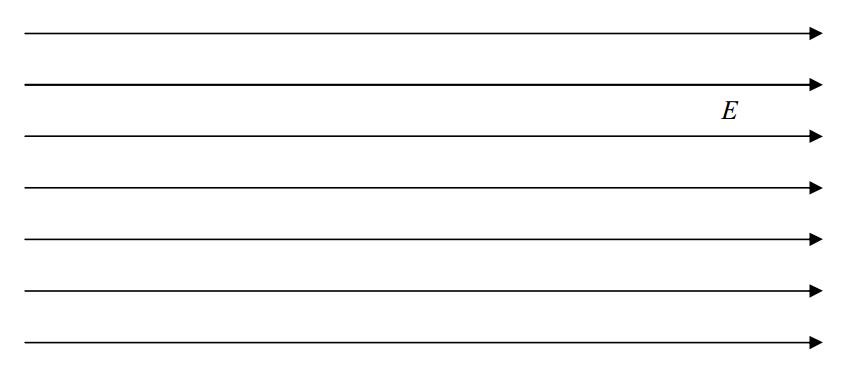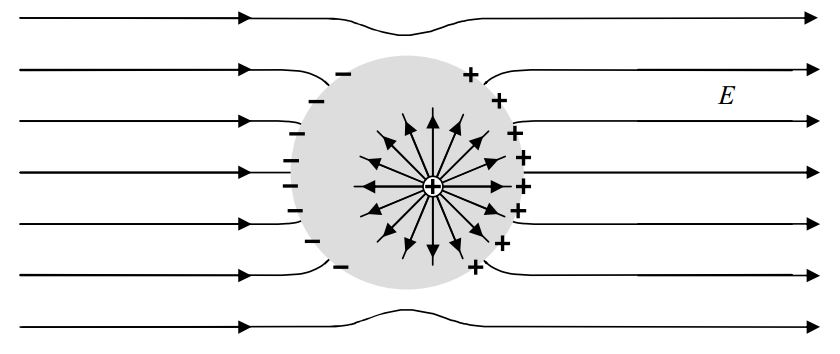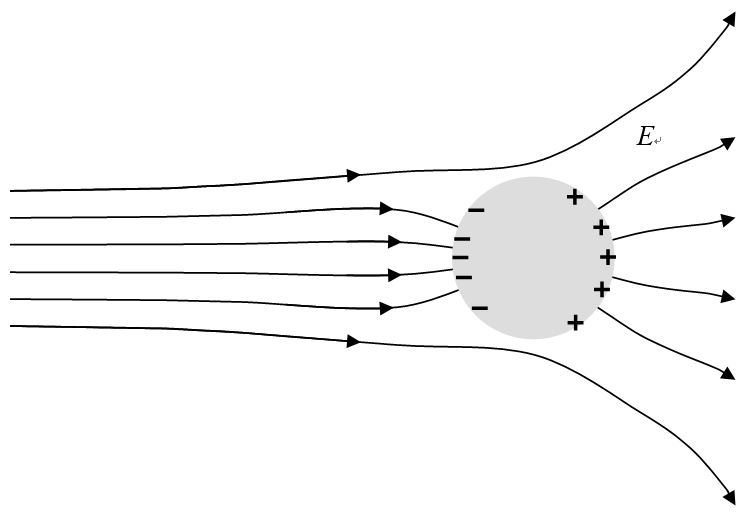$$\require{cancel}$$

# B4: Conductors and the Electric Field

An ideal conductor is chock full of charged particles that are perfectly free to move around within the conductor. Like all macroscopic samples of material, an ideal conductor consists of a huge amount of positive charge, and, when neutral, the same amount of negative charge. When not neutral, there is a tiny fractional imbalance one way or the other. In an ideal conductor, some appreciable fraction of the charge is completely free to move around within the conducting material. The ideal (perfect) conductor is well approximated by some materials familiar to you, in particular, metals. In some materials, it is positive charge that is free to move about, in some, it is negative, and in others, it is both. For our purposes, the observable effects of positive charge moving in one direction are so close to being indistinguishable from negative charge moving in the opposite direction that, we will typically treat the charge carriers as being positive without concern for what the actual charge carriers are.

Here, we make one point about conductors by means of an analogy. The analogy involves a lake full of fish. Let the lake represent the conductor and the fish the charge carriers. The fish are free to move around anywhere within the lake, but, and this is the point, they can’t, under ordinary circumstances, escape the lake. They can go to every boundary of the body of water, you might even see some on the surface, but, they cannot leave the water. This is similar to the charge carriers in a conductor surrounded by vacuum or an insulating medium such as air. The charges can go everywhere in and on the conductor, but, they cannot leave the conductor.

The facts we have presented on the nature of charge, electric fields, and conductors allow one to draw some definite conclusions about the electric field and unbalanced charge within the material of, and at or on the surface of, an ideal conductor. Please try to reason out the answers to the following questions:

1. Suppose you put a neutral ideal conducting solid sphere in a region of space in which there is, initially, a uniform electric field. Describe (as specifically as possible) the electric field inside the conductor and the electric field at the surface of the conductor. Describe the distribution of charge in and on the conductor.
2. Repeat question 1 for the case of a non-uniform field.
3. Suppose you put some charge on an initially-neutral, solid, perfectly-conducting sphere (where the sphere is not in a pre-existing electric field). Describe the electric field inside the conductor, at the surface of the conductor, and outside the conductor as a result of the unbalanced charge. Describe the distribution of the charge in and on the conductor.
4. Repeat questions 1-3 for the case of a hollow perfectly-conducting spherical shell (with the interior being vacuum).
5. How would your answers to questions 1-4 change if the conductor had some shape other than spherical?

Here we provide the answers (preceded in each case, with the corresponding question).

1) Suppose you put a neutral ideal conducting solid sphere in a region of space in which there is, initially, a uniform electric field. Describe (as specifically as possible) the electric field inside the conductor and the electric field at the surface of the conductor. Describe the distribution of charge in and on the conductor.We put a solid, ideal conductor in it. The electric field permeates everything, including the conductor.The charged particles in the conductor respond to the force exerted on them by the electric field. (The force causes acceleration, the acceleration of particles that are initially at rest causes them to acquire some velocity. In short, they move.) All this occurs in less than a microsecond. The net effect is a redistribution of the charged particles.Now, get this! The charged particles create their own electric field.The total electric field at any point in the conductor is the vector sum of the original electric field and the electric field due to the redistributed charged particles. Since they are oppositely directed, the two contributions to the electric field inside the conductor tend to cancel each other. Now comes the profound part of the argument: the two contributions to the electric field at any point in the conductor exactly cancel. We know they have to completely cancel because, if they didn’t, the free-to-move-charge in the conductor would move as a result of the force exerted on it by the electric field. And the force on the charge is always in a direction that causes the charge to be redistributed to positions in which it will create its own electric field that tends to cancel the electric field that caused the charge to move. The point is that the charge will not stop responding to the electric field until the net electric field at every point in the conductor is zero.

So far, in answer to the question, we have: The electric field is zero at all points inside the conductor, and, while the total charge is still zero, the charge has been redistributed as in the following diagram:Recall that we were also called upon to describe the electric field at the surface of the conductor. Note that the charge on the surface of the sphere will not only contribute to the electric field inside the conductor, it will also contribute to the electric field outside. The net effect of all the contributions to the electric field in the near vicinity of the sphere is to cause the electric field to be normal to (perpendicular to) the surface of the sphere at all points where it meets the sphere.How is it that we are able to assert this without doing any calculations? Here’s the argument: If the electric field at the surface had a component parallel to the surface, then the charged particles on the surface of the conductor would experience a force directed along the surface. Since those particles are free to move anywhere in the conductor, they would be redistributed. In their new positions, they would make their own contribution to the electric field in the surface and their contribution would cancel the electric field that caused the charge redistribution.

About the charge distribution: The object started out neutral and no charge has left or entered the conductor from the outside world so it is still neutral. But we do see a separation of the two different kinds of charge. Something that we have depicted but not discussed is the assertion that all the charge resides on the surface. (In the picture above, there is positive charge on the right surface of the sphere and an equal amount of negative charge on the left side.) How do we know that all charge must be on the surface? Assume that there was a positive point charge at some location within the conductor:The electric field of that point charge would cause the free-to-move charge in the conductor to move, and it would keep moving as long as there was an electric field. So where would the charge move in order to cancel out the electric field of the positive point charge. You can try any arrangement of charge that you want to, around that positive point charge, but, if it is stipulated that there be a net positive charge at that location, there is no way to cancel out the electric field of that positive charge. So the situation doesn’t even occur. If it did happen, the particle would repel the conductor’s free-to-move-positive charge away from the stipulated positive charge, so that (excluding the stipulated positive charge under consideration) the conductor would have a net negative charge at that location, an amount of negative charge exactly equal to the originally stipulated positive charge. Taking the positive charge into account as well, the point, after the redistribution of charge, would be neutral. The point of our argument is that, under static conditions, there can be no net charge inside the material of a perfect conductor. Even if you assume there to be some, it would soon be neutralized by the nearly instantaneous charge redistribution that it would cause.

Next question:

2) Repeat question 1 for the case of a non-uniform field. (Question 1 asked for a description of the charge distribution that develops on a solid neutral conducting sphere when you place it in a uniform electric field.)

Answer: Here is a depiction of an example of a non-uniform field:If we put a solid, perfectly-conducting sphere in it we get:The same arguments lead to the same conclusions. When, after less than a microsecond, the new static conditions are achieved: There can be no electric field inside the conductor or else the free-to-move-charges in it would still be moving around within the volume of the conductor. There can be no unbalanced charge within the volume of the conductor or else there would be an electric field inside the conductor. Hence, any locally unbalanced charge (overall, the initially neutral sphere remains neutral) must be on the surface. The electric field has to be normal to the surface of the sphere or else the free-to-move-charge at the surface would still be moving around on the surface. The only thing that is different in this case, as compared to the initially-uniform electric field case, is the way the charge is distributed on the surface. We see that the negative charge is more bunched up than the positive charge in the case at hand. In the initially-uniform electric field case, the positive charge distribution was the mirror image of the negative charge distribution.

Next Question:

3) Suppose you put some charge on an initially-neutral, solid, perfectly-conducting sphere (where the sphere is not in a pre-existing electric field). Describe the electric field inside the conductor, at the surface of the conductor, and outside the conductor as a result of the unbalanced charge. Describe the distribution of the charge in and on the conductor.

Again, we assume that we have waited long enough (less than a microsecond) for static conditions to have been achieved. There can be no charge within the bulk of the conductor or else there would be an electric field in the conductor and there can’t be an electric field in the conductor or else the conductor’s free-to-move charge would move and static conditions would not be prevailing. So, all the unbalanced charge must be on the surface. It can’t be bunched up more at any location on the surface than it is at any other location on the surface or else the charge on the edge of the bunch would be repelled by the bunch and it would move, again in violation of our stipulation that we have waited until charge stopped moving. So, the charge must be distributed uniformly over the surface of the sphere. Inside the sphere there is no electric field. Where the outside electric field meets the surface of the sphere, the electric field must be normal to the surface of the sphere. Otherwise, the electric field at the surface would have a vector component parallel to the surface which would cause charge to move along the surface, again in violation of our static conditions stipulations. Now, electric field lines that are perpendicular to the surface of a sphere lie on lines that pass through the center of the sphere. Hence, outside the sphere, the electric field lines form the same pattern as the pattern that would be formed by a point charge at the location of the center of the sphere (with the sphere gone). Furthermore, if you go so far away from the sphere that the sphere “looks like” a point, the electric field will be the same as that due to a point charge at the location of the center of the sphere. Given that outside the sphere, it has the same pattern as the field due to a point charge at the center of the sphere, the only way it can match up with the point charge field at a great distance from the sphere, is if it is identical to the point charge field everywhere that it exists. So, outside the sphere, the electric field is indistinguishable from the electric field due to the same amount of charge that you put on the sphere, all concentrated at the location of the center of the sphere (with the sphere gone).Next question:

4) Repeat questions 1-3 for the case of a hollow perfectly-conducting spherical shell (with the interior being vacuum).

In all three cases we have considered so far, the interior of the sphere has played no role. It is initially neutral and it is neutral after the sphere is placed in a pre-existing electric field or some charge is placed on it. Nothing would change if we removed all that neutral material making up the bulk of the conductor, leaving nothing but a hollow shell of a sphere. Hence all the results that we found for the solid sphere apply to the hollow sphere. In particular, the electric field at all points inside an empty hollow perfectly-conducting spherical shell is, under all conditions, zero.

Last question:

5) How would your answers to questions 1-4 change if the conductor had some shape other than spherical?

For a solid perfect conductor, the electric field and the charge everywhere inside would have to be zero for the same reasons discussed above. Furthermore, the electric field would have to be normal to the surface for the same reasons as before. Again, it would not make any difference if we hollow out the conductor by removing a bunch of neutral material. The only things that would be different for a non-spherical conductor are the way the charge would be distributed on the surface, and, the outside electric field. In particular, if you put some charge on a perfectlyconducting object that is not a sphere, the electric field in the vicinity of the object will not be the same as the electric field due to a point charge at the center of the object (although the difference would be negligible at great enough distances from the object).